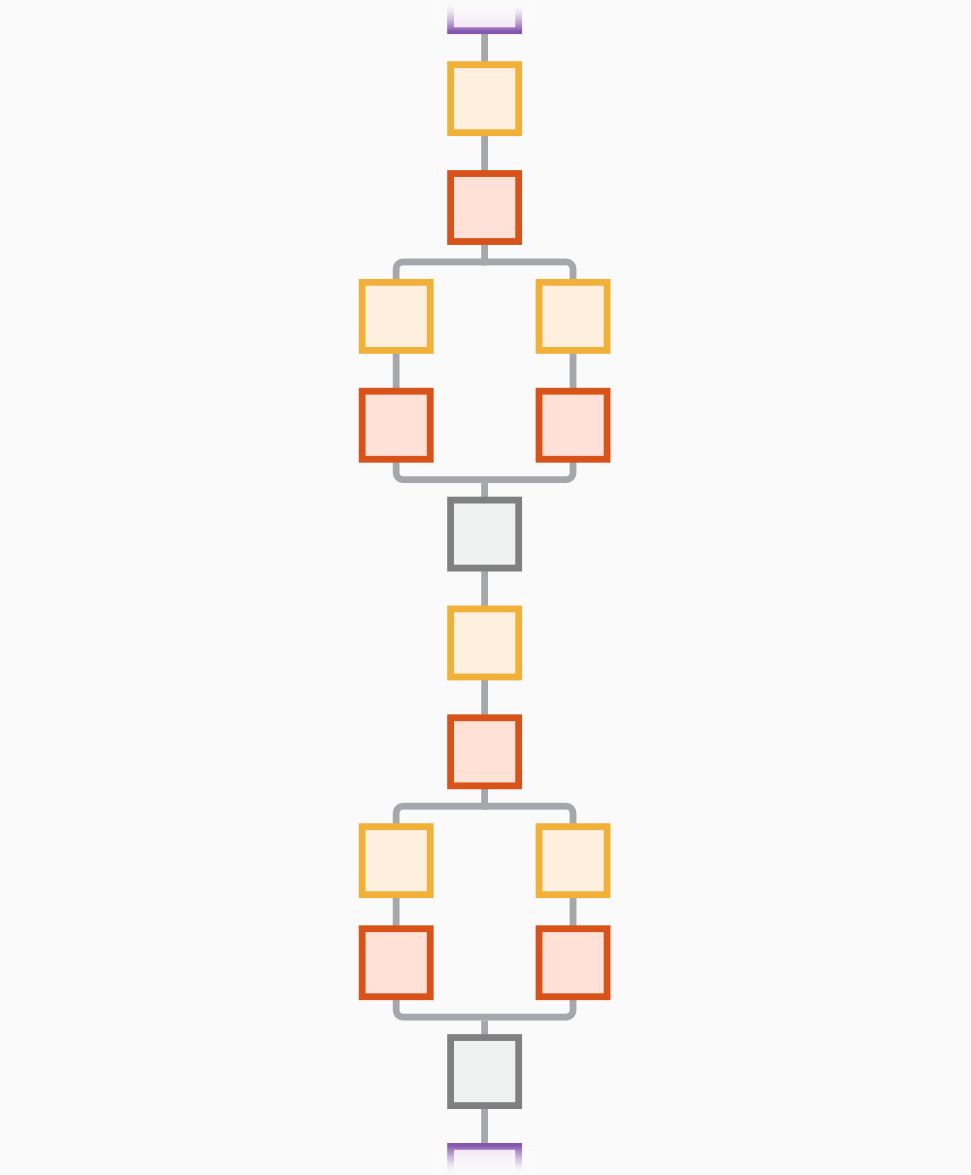# squeezenet

SqueezeNet 卷积神经网络

•## 语法

``net = squeezenet``
``net = squeezenet('Weights','imagenet')``
``lgraph = squeezenet('Weights','none')``

## 说明

SqueezeNet 是深度为 18 层的卷积神经网络。您可以从 ImageNet 数据库  中加载该网络的预训练版本，该版本基于 ImageNet 数据库的超过一百万个图像进行训练。该预训练网络可以将图像分类至 1000 个目标类别（例如键盘、鼠标、铅笔和多种动物）。因此，该网络已基于大量图像学习了丰富的特征表示。此函数返回一个 SqueezeNet v1.1 网络，它具有与 SqueezeNet v1.0 相似的准确度，但每次预测需要更少的浮点运算 。该网络的图像输入大小为 227×227。有关 MATLAB® 中预训练网络的详细信息，请参阅预训练的深度神经网络

``net = squeezenet` 返回基于 ImageNet 数据集训练的 SqueezeNet 网络。 `
``net = squeezenet('Weights','imagenet')` 返回基于 ImageNet 数据集训练的 SqueezeNet 网络。此语法等效于 `net = squeezenet`。`
``lgraph = squeezenet('Weights','none')` 返回未经训练的 SqueezeNet 网络架构。`

## 示例

`net = squeezenet`
```net = DAGNetwork with properties: Layers: [68×1 nnet.cnn.layer.Layer] Connections: [75×2 table]```

SqueezeNet 包含在 Deep Learning Toolbox™ 中。要加载其他网络，请使用 `googlenet` 等函数来获取链接，以便从附加功能资源管理器下载预训练网络。

`unzip("MerchData.zip");`

`deepNetworkDesigner(squeezenet);`• 将初始学习率设置为较小的值以减慢迁移的层中的学习速度。

• 指定验证频率，以便每经过一轮训练就计算一次基于验证数据的准确度。

• 指定少量轮数。一轮训练是对整个训练数据集的一个完整训练周期。对于迁移学习，所需的训练轮数相对较少。

• 指定小批量大小，即每次迭代中使用多少个图像。为了确保在每轮训练中都使用整个数据集，请设置小批量大小以均分训练样本的数量。`trainInfoStruct_1`
```trainInfoStruct_1 = struct with fields: TrainingLoss: [1×40 double] TrainingAccuracy: [1×40 double] ValidationLoss: [3.3420 NaN NaN NaN 2.1187 NaN NaN NaN NaN 1.4291 NaN NaN NaN NaN 0.8527 NaN NaN NaN NaN 0.5849 NaN NaN NaN NaN 0.4678 NaN NaN NaN NaN 0.3967 NaN NaN NaN NaN 0.3875 NaN NaN NaN NaN 0.3749] ValidationAccuracy: [20 NaN NaN NaN 30 NaN NaN NaN NaN 55.0000 NaN NaN NaN NaN 65 NaN NaN NaN NaN 85 NaN NaN NaN NaN 95 NaN NaN NaN NaN 95 NaN NaN NaN NaN 95 NaN NaN NaN NaN 95] BaseLearnRate: [1×40 double] FinalValidationLoss: 0.3749 FinalValidationAccuracy: 95 ```

`I = imread("MerchDataTest.jpg");``I = imresize(I, [227 227]);`

```[YPred,probs] = classify(trainedNetwork_1,I); imshow(I) label = YPred; title(string(label) + ", " + num2str(100*max(probs),3) + "%");```SqueezeNet 已基于超过一百万个图像进行训练，可以将图像分为 1000 个对象类别（例如键盘、咖啡杯、铅笔和多种动物）。该网络已基于大量图像学习了丰富的特征表示。网络以图像作为输入，然后输出图像中对象的标签以及每个对象类别的概率。```unzip('MerchData.zip'); imds = imageDatastore('MerchData', ... 'IncludeSubfolders',true, ... 'LabelSource','foldernames');```

`[imdsTrain,imdsValidation] = splitEachLabel(imds,0.7,'randomized');`

```numTrainImages = numel(imdsTrain.Labels); idx = randperm(numTrainImages,16); I = imtile(imds, 'Frames', idx); figure imshow(I)````net = squeezenet;`

`analyzeNetwork(net)``inputSize = net.Layers(1).InputSize`
```inputSize = 1×3 227 227 3 ```

`lgraph = layerGraph(net); `

```[learnableLayer,classLayer] = findLayersToReplace(lgraph); [learnableLayer,classLayer] ```
```ans = 1x2 Layer array with layers: 1 'conv10' 2-D Convolution 1000 1x1x512 convolutions with stride [1 1] and padding [0 0 0 0] 2 'ClassificationLayer_predictions' Classification Output crossentropyex with 'tench' and 999 other classes ```

`numClasses = numel(categories(imdsTrain.Labels))`
```numClasses = 5 ```
```newConvLayer = convolution2dLayer([1, 1],numClasses,'WeightLearnRateFactor',10,'BiasLearnRateFactor',10,"Name",'new_conv'); lgraph = replaceLayer(lgraph,'conv10',newConvLayer);```

```newClassificatonLayer = classificationLayer('Name','new_classoutput'); lgraph = replaceLayer(lgraph,'ClassificationLayer_predictions',newClassificatonLayer);```

```pixelRange = [-30 30]; imageAugmenter = imageDataAugmenter( ... 'RandXReflection',true, ... 'RandXTranslation',pixelRange, ... 'RandYTranslation',pixelRange); augimdsTrain = augmentedImageDatastore(inputSize(1:2),imdsTrain, ... 'DataAugmentation',imageAugmenter);```

`augimdsValidation = augmentedImageDatastore(inputSize(1:2),imdsValidation);`

```options = trainingOptions('sgdm', ... 'MiniBatchSize',11, ... 'MaxEpochs',7, ... 'InitialLearnRate',2e-4, ... 'Shuffle','every-epoch', ... 'ValidationData',augimdsValidation, ... 'ValidationFrequency',3, ... 'Verbose',false, ... 'Plots','training-progress');```

`netTransfer = trainNetwork(augimdsTrain,lgraph,options);``[YPred,scores] = classify(netTransfer,augimdsValidation);`

```idx = randperm(numel(imdsValidation.Files),4); figure for i = 1:4 subplot(2,2,i) I = readimage(imdsValidation,idx(i)); imshow(I) label = YPred(idx(i)); title(string(label)); end``````YValidation = imdsValidation.Labels; accuracy = mean(YPred == YValidation)```
```accuracy = 1 ```

`net = squeezenet;`

```I = imread('peppers.png'); figure imshow(I)````sz = net.Layers(1).InputSize`
```sz = 1×3 227 227 3 ```

```I = imresize(I,sz(1:2)); figure imshow(I)````label = classify(net,I)`
```label = categorical bell pepper ```

```figure imshow(I) title(label)``````unzip("MerchData.zip"); imds = imageDatastore("MerchData", ... IncludeSubfolders=true, ... LabelSource="foldernames"); [imdsTrain,imdsTest] = splitEachLabel(imds,0.7,"randomized");```

```numImagesTrain = numel(imdsTrain.Labels); idx = randperm(numImagesTrain,16); I = imtile(imds,"Frames",idx); figure imshow(I)````net = squeezenet;`

`analyzeNetwork(net)``inputSize = net.Layers(1).InputSize`
```inputSize = 1×3 227 227 3 ```

```augimdsTrain = augmentedImageDatastore(inputSize(1:2),imdsTrain); augimdsTest = augmentedImageDatastore(inputSize(1:2),imdsTest); layer = "pool10"; featuresTrain = activations(net,augimdsTrain,layer,OutputAs="rows"); featuresTest = activations(net,augimdsTest,layer,OutputAs="rows");```

```TTrain = imdsTrain.Labels; TTest = imdsTest.Labels;```

`mdl = fitcecoc(featuresTrain,TTrain);`

`YPred = predict(mdl,featuresTest);`

```idx = [1 5 10 15]; figure for i = 1:numel(idx) subplot(2,2,i) I = readimage(imdsTest,idx(i)); label = YPred(idx(i)); imshow(I) title(label) end````accuracy = mean(YPred == TTest)`
```accuracy = 0.9500 ```

## 输出参数

 ImageNet. http://www.image-net.org

 Iandola, Forrest N., Song Han, Matthew W. Moskewicz, Khalid Ashraf, William J. Dally, and Kurt Keutzer. "SqueezeNet: AlexNet-level accuracy with 50x fewer parameters and <0.5 MB model size." Preprint, submitted November 4, 2016. https://arxiv.org/abs/1602.07360.

 Iandola, Forrest N. "SqueezeNet." https://github.com/forresti/SqueezeNet.